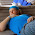## Friday, 16 March 2007

### The Java code 5 results per line solution is...?for (values = startvalue; values <= endvalue; values++) {
if (count == 5)
{
System.out.println( + values + ", ");
count = 1;
}
else
{
System.out.print( +values + ", ");
count = count + 1;
}
}

Many thanks to my tutor Prathmesh!

#### 1 comment:

1.A simpler solution is:

for (values = startvalue; values <= endvalue; values++) {
System.out.print(values + ", ");
count++;
if (count == 5)
{
System.out.print("\n");
count = 1;
}
}

Not tested though. Should anything be wrong I would guess count++; is the wrong side of the IF check... if it is printing 4 entries per line (Or 6 for that matter) try putting count++; after the IF check instead of before it :)